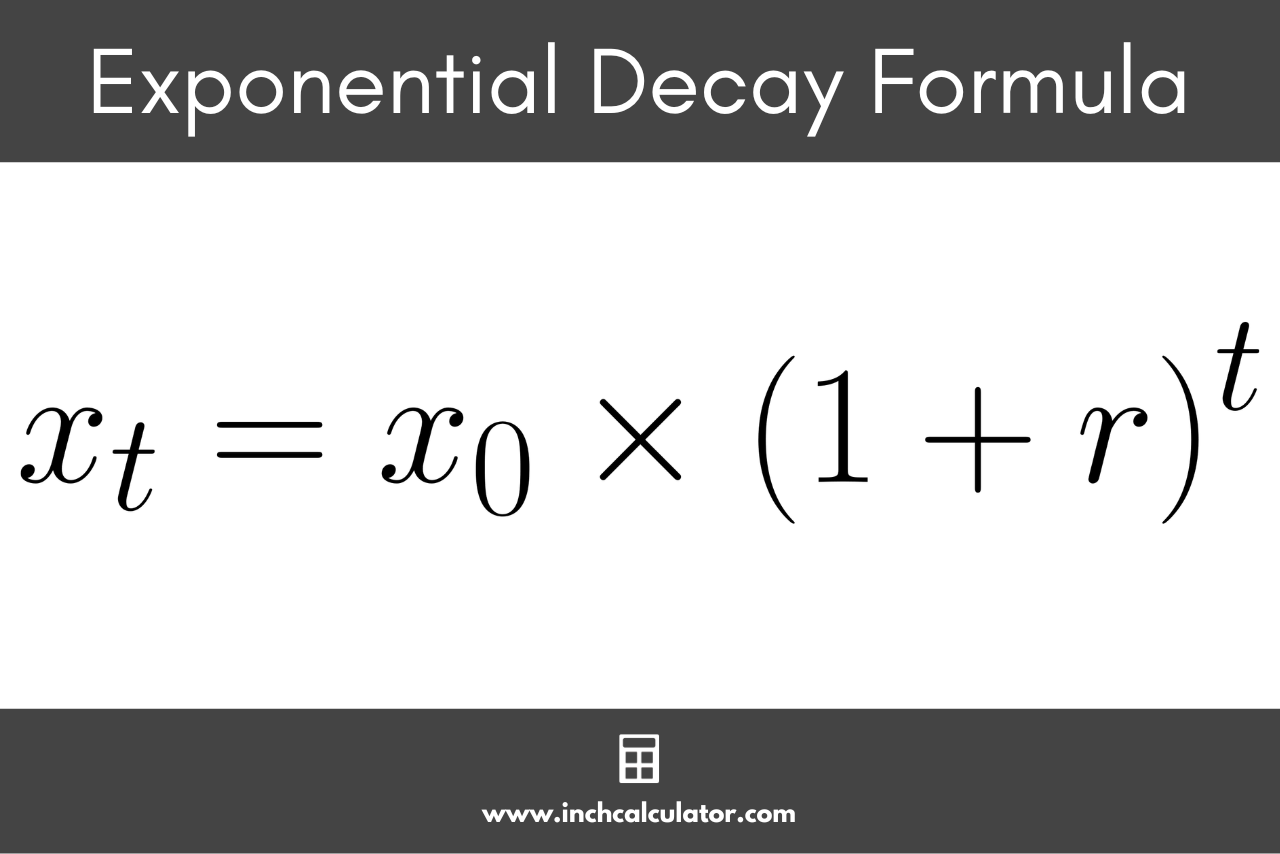# Exponential Decay Calculator

Use our exponential decay calculator to find reduction in value over time.

%

## Final Value:

xt =

Learn how we calculated this below

## How to Calculate Exponential Decay

Exponential decay is the reduction in a value by the same percentage over a period of time, where the percentage of decay during each period includes the value of decay from the previous periods.

Exponential decay is the mechanism behind things such as half-life decay.

Exponential decay is the inverse of exponential growth, and you can calculate it using a formula.

### Exponential Decay Formula

The formula to find a value after exponential decay is:

x_{t}=x_{0} \times \left ( 1 + r \right ) ^{t}

The final value xt is equal to the initial value x0 times 1 plus the decay rate r raised to the power of the number of time periods t.For example, let’s calculate the decay in a value of 250 over 5 time periods given a decay rate of 12%.

x_{t}=250 \times \left ( 1 + -0.12 \right ) ^{5}
x_{t}=250 \times 0.88^{5}
x_{t}=250 \times 0.5277
x_{t}=131.93

So, a value of 250 that decays at a rate of 12% over 5 time periods will be 131.93.